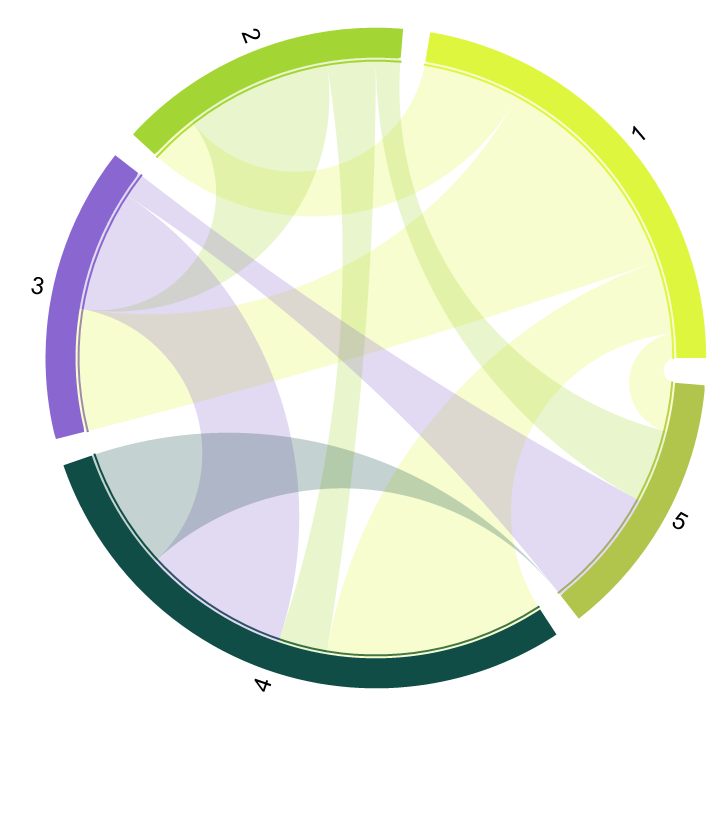#Function Repository Resource:

# ChordDiagram

Make a weighted connectivity graph using circular embedding

Contributed by: Georgios Varnavides
 ResourceFunction["ChordDiagram"][graph] generates a chord diagram using a weighted graph.

## Details and Options

ResourceFunction["ChordDiagram"] requires an edge-weighted graph as input.
ResourceFunction["ChordDiagram"] draws each vertex of the weighted graph as a wedge, with width proportional to its WeightedAdjacencyMatrix row total.
ResourceFunction["ChordDiagram"] draws a ribbon made from two Poincaré arcs for each directed edge.
The following options can be specified:
 "Labels" choose the vertex labels "Colors" choose the ribbon colors for each edge "BackgroundOpacity" 0.25 set the background ribbon opacity "Interactive" include interactive mouseover "TrimEdges" 0 trim edges with small weights
Use "TrimEdges"n to avoid multiple thin ribbons in the resulting graphic.
"Labels" Automatic uses the vertex labels given by VertexList[graph].
"Colors" Automatic uses random colors.

## Examples

### Basic Examples

A chord diagram with a simple weighted graph:

 In:=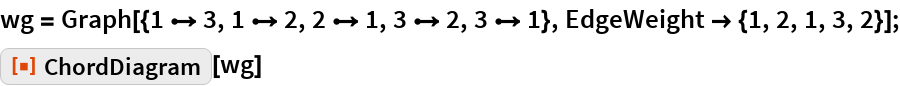Out=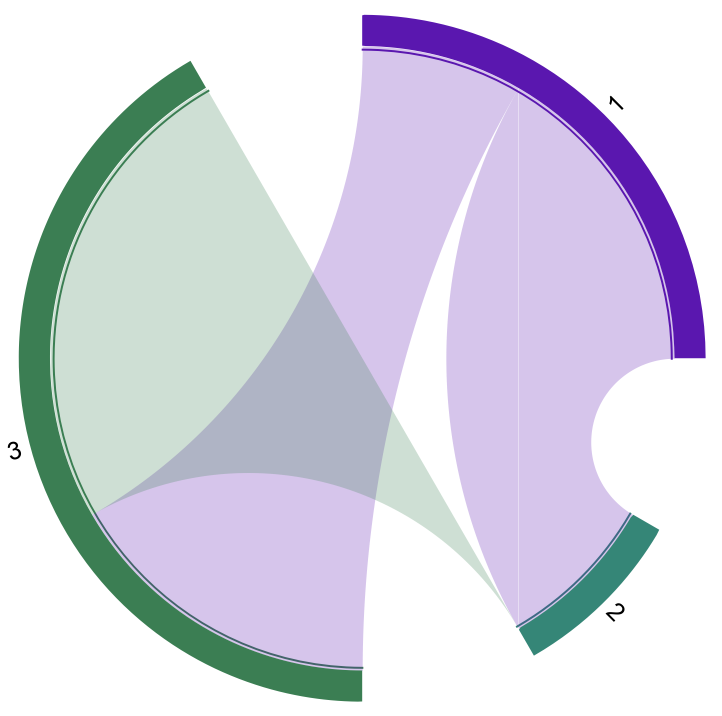### Options

#### Labels

By default, ChordDiagram uses VertexList as labels:

 In:=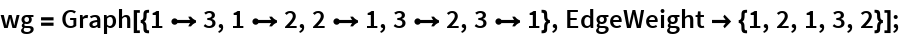In:=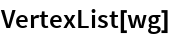Out=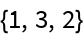In:=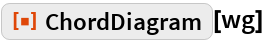Out=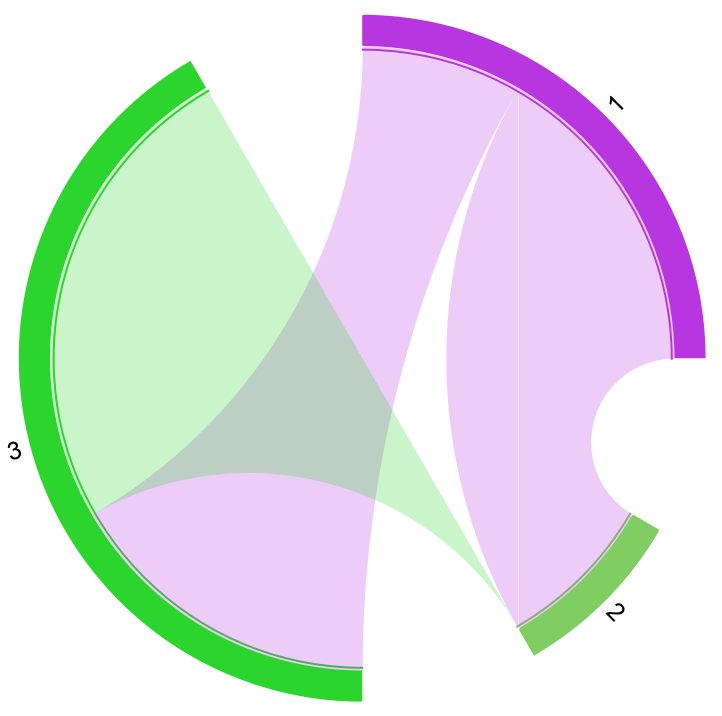Use an explicit list of strings to overwrite this:

 In:=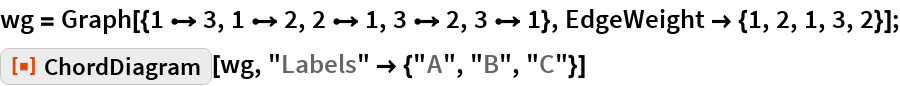Out=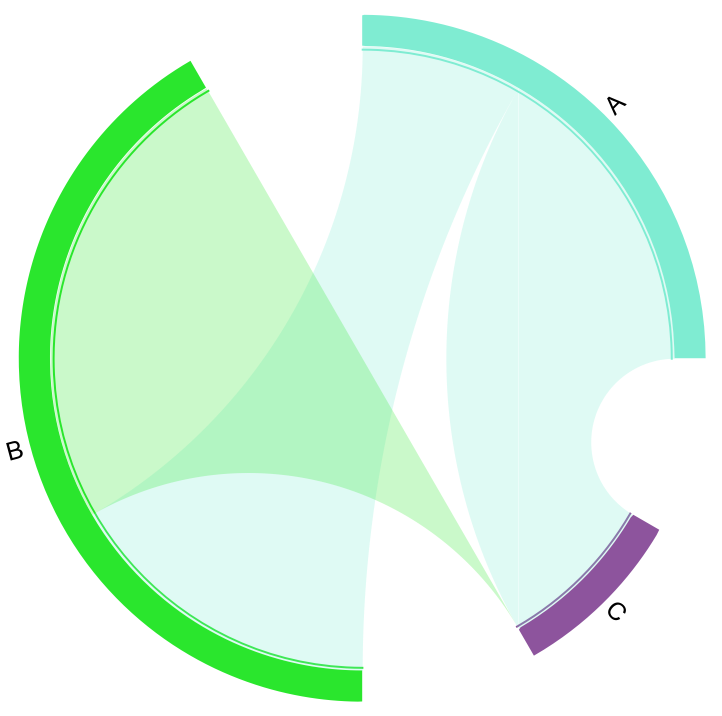#### TrimEdges

For realistic datasets, many edges may suffer from small weights:

 In:=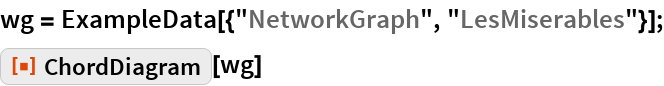Out=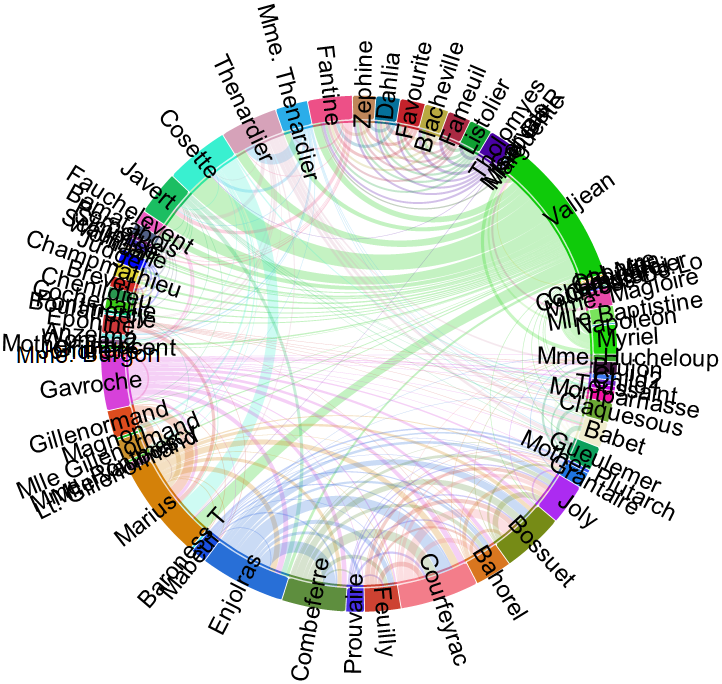Use "TrimEdges" to drop the lowest edge weights:

 In:=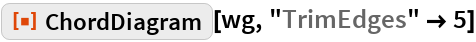Out=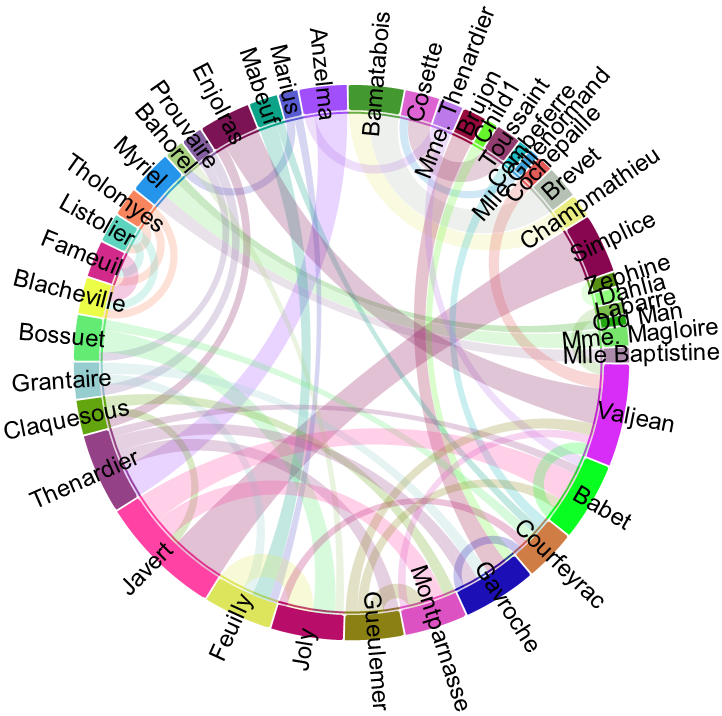#### Interactive

 In:=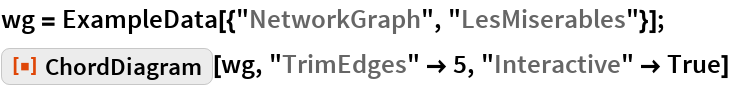Out=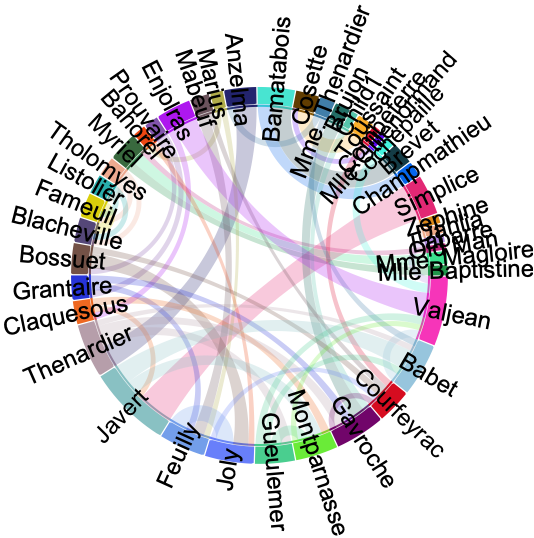### Applications

Use a directed graph to show asymmetric ribbon widths:

 In:=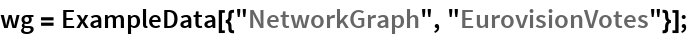In:=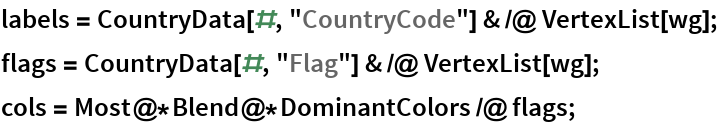In:=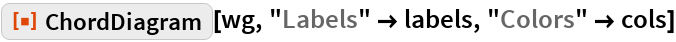Out=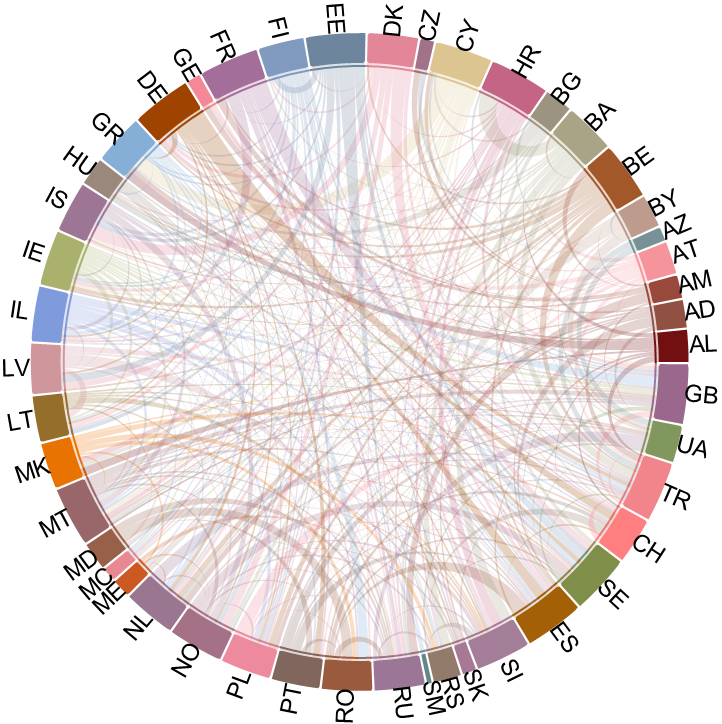### Properties and Relations

By default, the function accepts a weighted graph. If you instead have a weighted adjacency matrix, this can be converted to a weighted graph first:

 In:=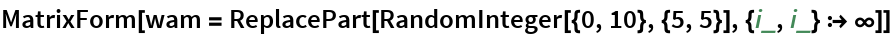Out=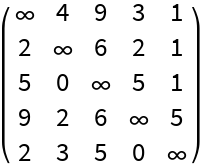In:=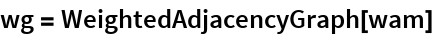Out=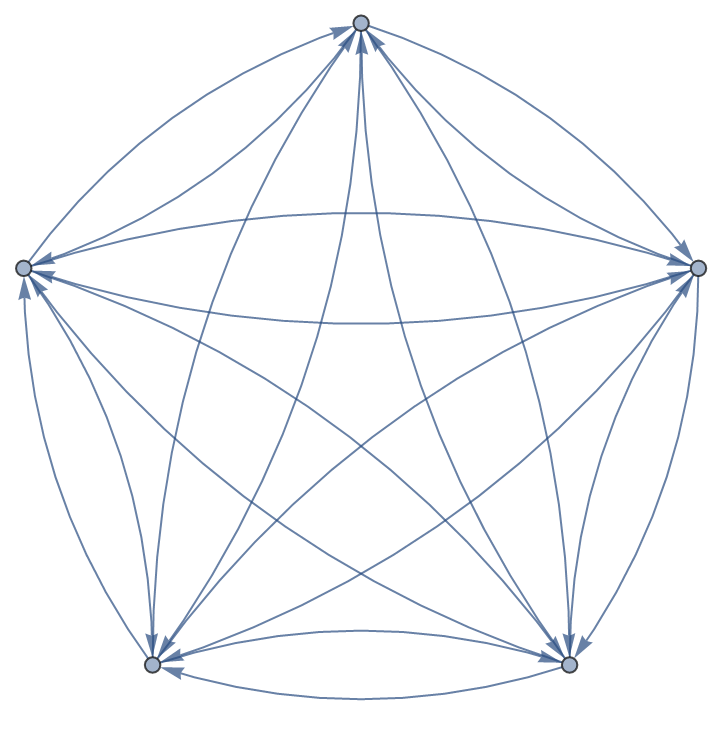In:=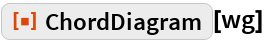Out=